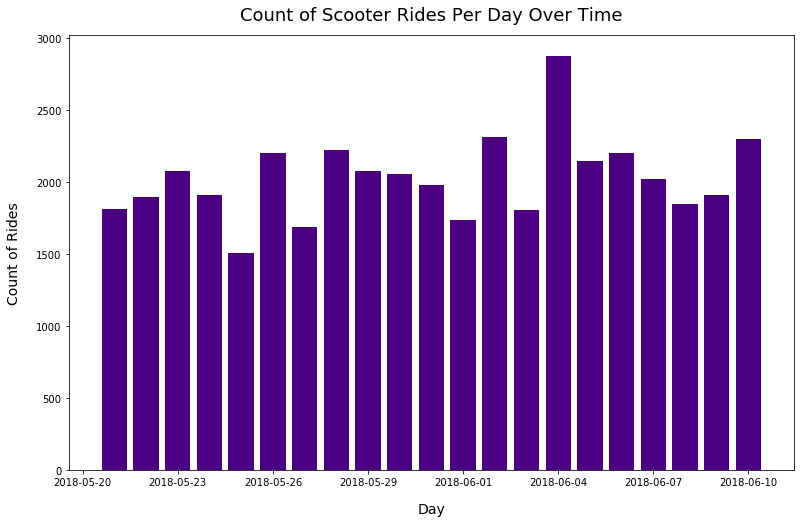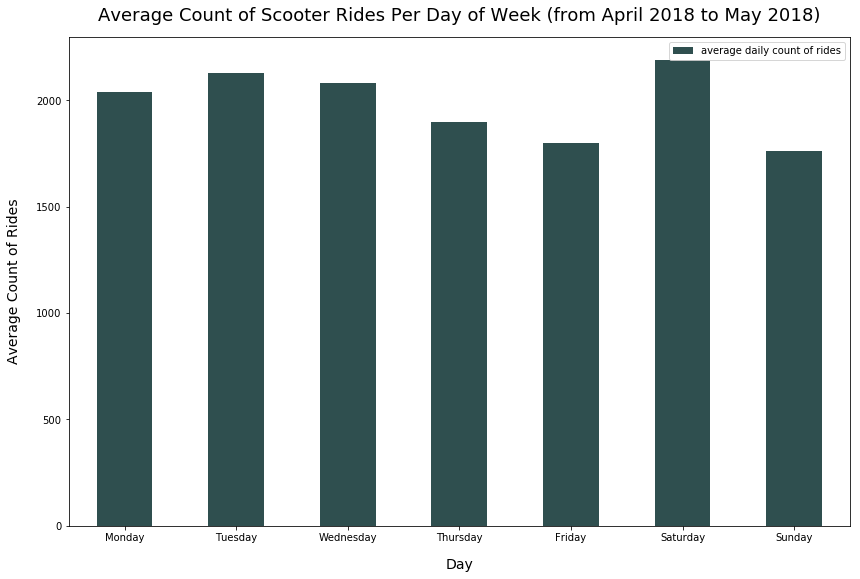Data Visualizations Best Practices Tutorial

# When to Use a Vertical Bar Chart

Vertical bar charts illustrate sizes of data using different bar heights.

For example, let's say we had a service that rented out scooters in San Francisco, California. Each day, we determine the count of total rides. We can plot the count of rides over the past 21 days, with the count each day being a bar of a certain height, to visualize the trend of rides recently.

I'll showcase a few examples of vertical bar charts below.

### Import Modules¶

In :
import pandas as pd
import matplotlib.pyplot as plt
%matplotlib inline


### Example: Scooter Rides Per Day Over Time¶

To continue with the example above, below is a sample of the original data collected for each ride.

Date Miles Ridden
6/9/2018 2.1
6/10/2018 1.5
6/10/2018 3.9

In order to get the count of rides each day, we'd have to perform a group by operation to group the data above by day, and for each day, get the count of rides.

#### Generate Daily Scooter Ride Data¶

In :
list_of_days = pd.date_range(start='5-21-2018', end='6-10-2018', freq='D')
count_rides = [1812, 1895, 2080, 1910, 1510, 2200, 1685, 2223, 2080, 2056, 1977, 1738, 2315, 1810, 2880, 2150, 2205, 2020, 1850, 1910, 2301]


#### Plot Scooter Daily Ride Counts¶

We choose a bar chart below because the count from each day is a total amount value - so bars help us illustrate the significance of this total value each day.

Alternatively, you could use a line plot. However, I think a bar chart is best here.

In :
plt.figure(figsize=(13, 8))
plt.bar(list_of_days, count_rides, align='center', color='indigo')
plt.title("Count of Scooter Rides Per Day Over Time", fontsize=18, y=1.02)#### Explanation of Scooter Daily Ride Counts¶

There's no obvious trend from the visualization above. There looks to be a slight increase in daily ride count over time - but I wouldn't jump to that conclusion from this visualization above.

Based on the visualization above, I'd be interested to explore average daily ride counts per day of week (such as Monday versus Tuesday).

### Example: Average Rides Per Day¶

The visualization above was meant to illustrate a possible trend in ride counts per day over time.

However, now I'm interested to explore the average number of rides per day of week. Perhaps more people ride on Mondays than Sundays because they likely use the scooters to commute.

Different than the visualization below, we'll now want a categorical value, the day of the week, on the x-axis, and the average count of rides on the y-axis.

#### Generate Data for Average Count of Scooter Rides Per Day¶

In :
days = ['Monday', 'Tuesday', 'Wednesday', 'Thursday', 'Friday', 'Saturday', 'Sunday']
avg_count_rides = [2040, 2130, 2080, 1900, 1800, 2190, 1760]


#### Plot Average Scooter Rides Per Day¶

I think a vertical bar plot works well here because the day names (ex - Monday) are short and fit well on the x-axis.

Also, we often view the days of a week as a progression from one day to the next, starting from Monday and ending on Sunday. Therefore, I think the chart below works well as a vertical bar chart in order of day of week rather than a horizontal bar chart.

In :
df = pd.DataFrame({'day': days, 'average daily count of rides': avg_count_rides})

In :
df.set_index('day').plot(kind='bar', color='darkslategray', figsize=(14, 9))
plt.xticks(rotation=0)
plt.ylabel("Average Count of Rides", fontsize=14, labelpad=15)